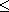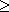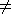Chapter 10 - If...

All the program we've seen so far have been pretty predictable - they went straight through doing all the instructions, & then maybe went back to the beginning again. This is not all that useful. In practice the computer would be expected to make decisions & act accordingly; it does this using the IF statement.

Clear the computer (using NEW), & type in & run this terribly amusing little program:

10 PRINT "SHALL I TELL YOU A JOKE?"

20 INPUT A\$

30 IF A\$="GET LOST" THEN GOTO 200

40 PRINT "HOW MANY LEGS HAS A HORSE GOT?"

50 INPUT LEGS

60 IF LEGS=6 THEN GOTO 100

70 PRINT "NO, 6, FORE LEGS IN FRONT","AND TWO BEHIND."

80 STOP

100 PRINT "YES",,"SHALL I TELL YOU IT AGAIN?"

110 GOTO 20

200 PRINT "ALL RIGHT, THEN, I WONT."

Before we discuss the IF statement, you should first look at the STOP statement in line 80: a STOP statement stops the execution of the program, giving report 9.

Now as you can see, an IF statement takes the form

IF condition THEN statement

The statements here are GOTO statements, but they could be anything at all, even more IF statements. The condition is something that is going to be worked out as either true or false; if it comes out as true then the statement after THEN is executed, but otherwise it is skipped over.

The most useful conditions compare two numbers or two strings: they can test whether two numbers are equal, or whether one is bigger than the other; & can test whether two strings are equal, or whether one comes before the other in alphabetical order. They use the relations =, <, >, <=, >= and <>.

=, which we have used twice in the program (once for numbers & once for strings) means 'equals'. It is not the same as the = in a LET statement.

< means 'is less than', so that

1<2

-2<-1

&      -3<1

are all true, but

1<0

&      0<-2

are false.

To see how this works, let us write a program to input numbers & display the biggest so far.

10 PRINT "NUMBER","BIGGEST SO FAR"

20 INPUT A

30 LET BIGGEST=A

40 PRINT A,BIGGEST

50 INPUT A

60 IF BIGGEST<A THEN LET BIGGEST=A

70 GOTO 40

The crucial part is line 60, which updates BIGGEST if its old value was smaller than the new input number A.

> (shifted M) means 'is greater than', & is just like < but the other way round. You can remember which is which, because the thin end points to the number that is supposed to be smaller.

<= (shifted R - do not type it as < followed by =) means 'is less than or equal to', so that it is like < except that it holds even if the two numbers are equal: this 2<=2 holds, but 2<2 does not.

>= (shifted Y) means 'is greater than or equal to' & is similarly like >.

<> (shifted T) means 'is not equal to' the opposite in meaning to =.

All six of these relational operations have priority 5.

Mathematicians usually write <=, >= and <> asand. They also write things like '2<3<4' to mean '2<3 and 3<4', but this is not possible in BASIC.

These relations can be combined using the logical operations AND, OR & NOT.

one relation AND another relation

is true whenever both relations are true.

one relation OR another

is true whenever one of the two relations is true (or both are).

NOT relation

is true whenever the relation is false and is false whenever the relation is true.

Logical expressions can be made with relations & AND, OR & NOT just as numerical expressions can be made with numbers & +, - and so on; you can even put in brackets if necessary. NOT has priority 4, AND 3 & OR 2.

Since (unlike other functions) NOT has fairly low priority, its argument does not need brackets unless it contains AND or OR; so NOT A = B means NOT (A = B) (which is the same as A <> B).

To illustrate this, clear the computer & try this program.

10 INPUT F\$

20 INPUT AGE

30 IF F\$="X" AND AGE<18 OR F\$="AA" AND AGE<14 THEN PRINT "DONT";

40 PRINT "LET IN."

50 GOTO 10

Here F\$ is supposed to be the category if a film - X for 18 years old & over, AA for 14 & over, & A or U for anyone, & the program works out whether a person of a given age is to be allowed to see the film.

Lastly, we can compare not only numbers, but also strings. We have seen '=' used in 'F\$="X"', & you can even use the other five, < & so on.

So what does 'less than' mean for strings? One thing it does not mean is 'shorter than', so don't make that mistake. We make the definition that one string is less than another if it comes first in alphabetical order: thus

 "SMITH" < "SMYTHE" "SMYTHE" > "SMITH" "BLOGGS" < "BLOGGS-BLACKBERRY" "BILLION" < "MILLION" "TCHAIKOVSKY" < "WAGNER" "DOLLAR" < "POUND"

all hold. <= means 'is less than or equal to', & so on, just as for numbers.

Note: In some versions of BASIC - but not on the ZX81 -, the IF statement can have the form

IF condition THEN line number

This means the same as

IF condition THEN GOTO line number

Summary

Statements: IF, STOP

Operations: =, <, >, <=, >=, <>, AND, OR

Function: NOT

Exercises

1. <> and = are opposites in the sense that NOT A=B is the same as A<>B

&

NOT A<>B is the same as A=B

Persuade yourself that >= is opposite to <, and <= is opposite to > so that you can always get rid of NOT from in front of a relation by changing the relation to its opposite.

Also,

NOT (a first logical expression AND a second)

is the same as

NOT (the first) OR NOT (the second),

&

NOT (a first logical expression OR a second)

is the same as

NOT (the first) AND NOT (the second).

Using this you can work NOTs through brackets until eventually they are all applied to relations, & then you can get rid of them. Thus, logically speaking, NOT is unnecessary. You might still find that using it makes a program clearer.

2. BASIC can sometimes work along different lines from English. Consider, for instance, the English clause 'if A doesn't equal B or C'. How would you write this in BASIC? [The answer is not

'IF A<>B OR C' nor 'IF A<>B OR A<>C']

Don't worry if you don't understand exercises 3, 4 & 5, the points covered in them are rather refined.

3. (For experts.)

Try

PRINT 1=2,1<>2

which you might expect to give a syntax error. In fact, as far as the computer is concerned, there is no such thing as a logical value.

(i) =, <, >, <=, >=, and <> are all number valued binary operations, with priority 5. The result is 1 (for true) if the relation holds, & 0 (for false) if it does not.

(ii) In

IF condition THEN statement

the condition can actually be any numeric expression. If its value is 0, then it counts as false, & any other value counts as true. This the IF statement means exactly the same as

IF condition <>0 THEN statement

(iii) AND, OR & NOT are also number valued operations.

 X AND Y has the value { X if Y is non-zero (counting as true) { 0 if Y is zero (counting as false) X OR Y has the value { 1 if Y is non-zero { X if Y is zero NOT X has the value { 0 if X is non-zero { 1 if X is zero

Read through the chapter again, in the light of this revelation, making sure that it all works.

In the expressions X AND Y, X OR Y & NOT X, X and Y will each usually take the value 0 or 1, for false or true. Work out the ten different combinations & check that they do what you expect AND, OR & NOT to do.

4. Try this program:

10 INPUT A

20 INPUT B

30 PRINT (A AND A>=B)+(B AND A<B)

40 GOTO 10

Each time it prints the larger of the two numbers A & B - why?

Convince yourself that you can think of

X AND Y

as meaning

'X if Y (else the result is 0)'

& of

X OR Y

as meaning

'X unless Y (in which case the result is 1)'

An expression using AND & OR like this is called a conditional expression. An example using OR could be

LET RETAIL PRICE=PRICE LESS VAT*(1.15 OR V\$="ZERO RATED")

Notice how AND tends to go with addition (because its default value is 0), & OR tends to go with multiplication (because its default value is 1).

5. You can also make string valued conditional expressions, but only using AND.

 X\$ AND Y\$ has the value { X\$ if Y is non-zero { if Y is zero

so it means 'X\$ if Y (else the empty string)'.

Try this program, which inputs two strings & puts them in alphabetical order.

10 INPUT A\$

20 INPUT B\$

30 IF A\$<=B\$ THEN GOTO 70

40 LET C\$=A\$

50 LET A\$=B\$

60 LET B\$=C\$

70 PRINT A\$;" ";("<" AND A\$<B\$)+("=" AND A\$=B\$);" ";B\$

80 GOTO 10

6. Try this program:

10 PRINT "X"

20 STOP

30 PRINT "Y"

When you run it, it will display "X" & stop with report 9/20. Now type

CONT

You might expect this to behave like 'GOTO 20', so that the computer would just stop again without displaying "Y"; but this would not be very useful, so things are arranged so that for reports with code (STOP statement executed), the line number is increased by 1 for a CONT statement. This in our example, 'CONT' behaves like 'GOTO 21' (which, since there are no lines between 20 & 30, behaves like 'GOTO 30').

7. Many versions of BASIC (but not the ZX81 BASIC) have an ON statement, which takes the form

ON numeric expression GOTO line number, line number,...,line number In this the numeric expression is evaluated; suppose its value is n then the effect is that of.

GOTO the nth line number

For instance

ON A GOTO 100, 200, 300, 400, 500

Here, if A has the value 2, then 'GOTO 200' is executed. In ZX81 BASIC this can be replaced by

GOTO 100*A

In case the line numbers don't go up neatly by hundreds like this, work out how you could use

GOTO a conditional expression